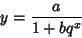## Logistic Growth Curve

The Population Growth law which arises frequently in biology and is given by the differential equation(1)

whereis the Malthusian Parameter andis the so-called Carrying Capacity (i.e., the maximum sustainable population). Rearranging and integrating both sides gives(2)(3)(4)

The curve(5)

is sometimes also known as the logical curve.

See also Gompertz Curve, Life Expectancy, Logistic Equation, Makeham Curve, Malthusian Parameter, Population Growth